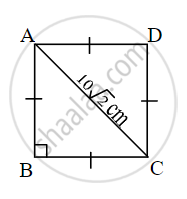# Choose the correct alternative: The diagonal of a square is 1012 cm then its perimeter is - Geometry

MCQ
Fill in the Blanks

Choose the correct alternative:

The diagonal of a square is 10sqrt(2) cm then its perimeter is ______

#### Options

• 10 cm

• 40 sqrt(2) cm

• 20 cm

• 40 cm

#### Solution

40 cmIn ∆ABC,

∠B = 90°, and ∠BAC = ∠BCA = 45°

∴ "AB" = 1/sqrt2 "AC" ...[Theorem of 45° – 45° – 90° triangle]

∴ "AB" = 1/sqrt2 xx 10sqrt2

∴ AB = 10 cm

∴ Perimeter of square= 4 (AB) = 4 × 10 = 40 cm.

Concept: Property of 30°- 60°- 90° Triangle Theorem
Is there an error in this question or solution?